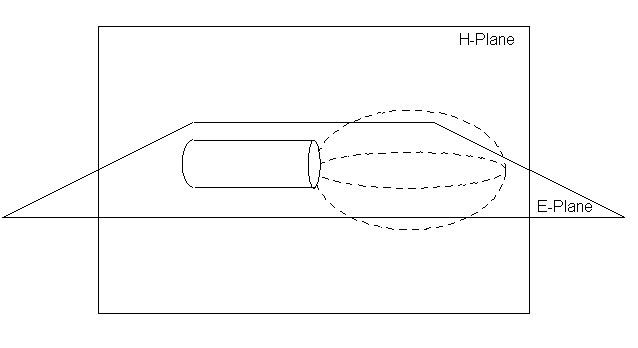# E plane and H plane

Updated on
Covid-19The E-plane and H-plane are reference planes for linearly polarized waveguides, antennas and other microwave devices.

## Contents

In waveguide systems, as in the electric circuits, it is often desirable to be able to split the circuit power into two or more fractions. In a waveguide system, an element called a junction is used for power division.

In a low frequency electrical network, it is possible to combine circuit elements in series or in parallel, thereby dividing the source power among several circuit components. In microwave circuits, a waveguide with three independent ports is called a TEE junction. The output of E-Plane Tee is 180° out of phase where the output of H-plane Tee is in phase.

## E-Plane

For a linearly-polarized antenna, this is the plane containing the electric field vector (sometimes called the E aperture) and the direction of maximum radiation. The electric field or "E" plane determines the polarization or orientation of the radio wave. For a vertically polarized antenna, the E-plane usually coincides with the vertical/elevation plane. For a horizontally polarized antenna, the E-Plane usually coincides with the horizontal/azimuth plane. E- plane and H-plane should be 90 degrees apart.

## H-plane

In the case of the same linearly polarized antenna, this is the plane containing the magnetic field vector (sometimes called the H aperture) and the direction of maximum radiation. The magnetizing field or "H" plane lies at a right angle to the "E" plane. For a vertically polarized antenna, the H-plane usually coincides with the horizontal/azimuth plane. For a horizontally polarized antenna, the H-plane usually coincides with the vertical/elevation plane.

## Co-Pol and Cross-Pol Directions

Co-polarization (Co-pol) on cross-polarization (X-pol) are defined for the radiating E and H planes. These directions are defined in spherical coordinates to correspond to the spherical wavefronts of the propagating wave. By convention the co-pol direction is the direction of the E field while the cross pol direction is the direction of the H field. Receive power for an co-pol oriented antenna is maximum while receive power is minimum for cross-pol orientation.

Similar Topics
Jamie Bryant
Phil Plait
Jack Fisher
Topics